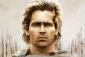# Power Functions

3 posts / 0 new
AlexanderPower Functions

If ax = b, by = c, and cx = a, prove that the product xyz = 1.

MONGOL○482

x=logab =(log b)/(log a)
y=logbc =(log c)/(log b)
z=logca =(log a)/(log c)

Jhun VertDo you mean cz = a? There is no z in your given.

Subscribe to MATHalino on